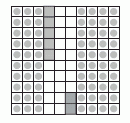Email us to get an instant 20% discount on highly effective K-12 Math & English kwizNET Programs!

#### Online Quiz (WorksheetABCD)

Questions Per Quiz = 2 4 6 8 10

### Grade 4 - Mathematics9.4 Decimal Problems

 Converting decimals to fractions Write the number without the decimal point. Divide the number with as many tens as the number of digits after the decimal point. Example: Convert 0.75 to a fraction. Numerator - 75 Denominator - 100 (since there are 2 numbers after the decimal point) Answer: 75/100 Adding decimals Add 0.35 and 0.42Take a square divide into hundred parts. Mark 0.35 by shading 3 tenths and colouring 5 hundreths. Similarly mark o.42 by shading 4 tenths and colouring 2 hundredths. Now count the total number of tenths and hundredths in the square. Answer: 0.77 Subtracting decimalsWrite the decimals one below the other as shown. Subtract as shown. Answer: 1.76 Directions: Answer the following questions. Also write at least 5 examples of your own for each of the following: * converting fraction to decimal * adding two decimals * subtracting two decimals
 Q 1: What is the sum of 32.8 + 53.6?85.486.286.485.5 Q 2: Which number is greater 2.35 or 2.262.352.26 Q 3: Fill in the blank 38.56 - 0.98 =36.5836.5037.58 Q 4: .5 =(fraction)5/10005/105/100 Q 5: Subtract the decimals 91.2 - 5.36 =85.8485.8083.88 Q 6: What decimal is between 9 and 10?9.38.910.511 Q 7: Subtract the decimals 8.08 - .88 =7.87.67.2 Q 8: Add the decimals 99.27 + .73 =100100010 Question 9: This question is available to subscribers only! Question 10: This question is available to subscribers only!